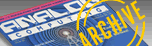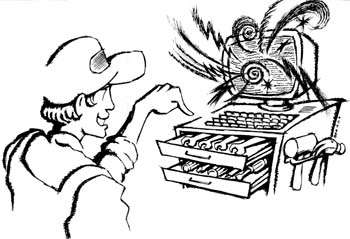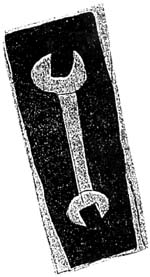`A.N.A.L.O.G. ISSUE 67 / DECEMBER 1988 / PAGE 38`

Action! Graphics Toolkit

by Monty McCartyAction! is a terrific language. It is fast, flexible and surprisingly easy to program in. Unfortunately, as you might have noticed with most other Atari programming languages, it comes up a little short on graphics routines other than the usual plot, DRAWTO and occasional player/missile commands. In an attempt to remedy this, and hopefully to get the ball rolling for more (and better) types of these routines to appear for all languages, here's the Action! Graphics Toolkit.

These routines reflect a bias of mine for working in high resolution, with speed being all important. To achieve this I used Clint Parker's fast graphics-eight plot routine from ANALOG #18. Also, for speed's sake, error trapping is at a bare minimum, so be careful.

Here are some general rules concerning the use of these routines. First, to see the demo program, just type in Listing 1 with your Action! cartridge (check your typing with D:CHECK in Action!) and save a copy before you compile and run it. To use the routines in your own programs, remove the procedure DEMO( ), since it is not needed. The variables declared at the beginning of the program should always be included. The screen buffer takes a big chunk of memory; so though there is enough memory for a fairly complex program, you will probably have to compile from disk.
In the descriptions below, the < plot > , < memory > and < text > after each command name tells you the command's type. The range of numbers for anything dealing with plot-type commands are 0-319 for X values and 1-192 for Y. The CX and CY parameters in a command such as BOX must always be larger than the X and Y parameters. The X and Y in such a command will always represent the upper left corner, and CX and CY will be the lower right corner.
The range for text and memory-type commands are 0-39 for X values and 0-191 for Y. Finally, use the COLOR variable normally to select plot colors 0 and 1.
Now, a short description of the commands and how to use them:

SCREEN(X,Y,CX,XY) - < plot > : This command defines the rectangular area of clipping for all commands which use the PLOT command. Nothing can be plotted outside of the area defined. This command does not work for any command dealing with text or moving blocks of memory.

UNCLIP( ) - < plot > : Simply returns the screen limits to the default values.

PLOT(X,Y) - < plot > : A very fast plot command slowed down somewhat by the addition of boundary error checking. You can now run a circle off the screen and not fear the dreaded Error 141, cursor out of range.

VLINE(Y,CY,X) - < plot > : A faster way to draw a straight vertical line than using DRAWTO. Y must be a smaller number than CY. X is the axis along which the line will be drawn.

HLINE(X,CX,Y) - < plot > : The horizontal version of the above, with similar restrictions.

DRAWTO (CX,CY) - < plot > : This will draw a line from the last point plotted to the point specified, slightly faster than the builtin DRAWTO. You can chain your DRAWTO's without PLOTs in between to create a series of lines.

CIRCLE(X,Y,R) - < plot > : Yes, this draws a circle. X and Y will be the center and R the radius. Remember, you can run circles off the screen or, using SCREEN, show only portions of circles, perhaps to create arcs.

BOX (X,Y,CX,CY) - < plot > : Draws unfilled rectangles.

FRAME( ) - < plot > : If you want to see the boundaries of the area you SCREENed off, FRAME will draw a border around it.

MOVE(T,F,L,M) - < memory > : The same as Action!'s MOVEBLOCK procedure except with the addition of being able to manipulate how the source memory is merged with the destination memory. As in MOVEBLOCK, T is for the destination address, F is for the origin address and L is for the number of bytes to move. If M equals one then the move works just like MOVEBLOCK. If it is two, then the source is ORed to the destination. A three means it will be ANDed and a four means it will be exclusive ORed. Check the Action! manual to find out what these mean, or better yet, try changing the options in the demo program and see for yourself.

CUT(X,Y,CX,CY) - < memory > : This will allow you to grab a block of screen memory and store it into a buffer for later use. In the demo program, the buffer, array CS, holds 1K of data. You can change it to any size your available memory can support. Remember, the screen is 40 bytes across by 192 lines down which equals 7680 bytes.

BLOCK(X,Y,CX,CY,F) - < memory > Makes a solid block. Useful for erasing areas quickly, special effects, etc. Since this is a memory-related command, its horizontal resolution is only 40, limiting its usefulness somewhat. The F (0-255) parameter, however, allows you to define the pattern made by the block, like colors and vertical patterns.

PASTE(X,Y,M) - < memory > : This puts whatever is in the CS buffer back into screen memory, and using the M parameter allows you the same options as MOVE to alter the result. Only the upper left (X,Y) corner need be provided. The rest is done for you.

PRINT8(ST, X,Y,SZ,M) - < text > : Writes text on the high-resolution screen in 24 (1-24) vertical sizes with the same M options as MOVE and PASTE. ST can be any byte, char or card array. X and Y is where the text will start and SZ is the vertical height-one is normal. In this case, M can be use to remove the white space surrounding the text or to create an inverse video effect. Check out the demo.

OPENW(X,Y,LN,LINES,SZ,N) - < text > Opens a window into which a text message is written. X and Y determines where the first line of text will start. LN is the length of the longest line of text in the window. LINES is the number of lines of text in the window. SZ is the size of the text and N determines if a frame will be made (1) or not (any other number). To set up a text window, first place your text in the card array, TW The first line of your text should be in the zero element (TW(0)="line one") of the array, the second line in the first element and so on, only one line per element. Determine your values for the above parameters, and that is pretty much all there is. The nice thing is that nothing written over by the text window will be harmed. Simply close the text window and it disappears, leaving your screen in its original state. You may have only one window open at a time and preserve the screen.

CLOSEW(D) - < text > : Closes the text window with the option to wait a predetermined amount of time. CLOSEW( ) is enough to just close the window. Since Action! is so fast, if you want a delay of any length, provide a fairly large number for D.

GWINDOW(X,Y,CX,CY,N) - < plot > Will create a solid-filled high-resolution box with or without a frame. If N equals one, a frame is drawn around the box, any other number means it won't.

 Some suggested uses     Here are some ideas to get you started. If for example, you are writing a paint program and need an undo feature, try this: Before a command that will alter the screen is executed, save the screen to the screen buffer (array BF) with the following command MOVEBLOCK(BF SC,7680); then to retrieve the screen to "undo" a mistake, MOVEBLOCK(SC,BF,7680) and the screen is back.     If you have a RAMdisk, you could replace the screen buffer by saving and retrieving the screen to the RAMdisk with bput and bget from the Action! toolkit. It is a little slower, but it works and saves 7680 bytes of program space. Bput and bget are also very useful for saving and loading screens to and from the disk drive.     It is possible to use CUT and PASTE to create simple motion or even animation. There is a simple example in the demo program using CUT, PASTE and MOVEBLOCK. I used MOVEBLOCK here since it is faster than MOVE.     If you have a Koalapad, Listing 2 gives an Action! routine for drawing with the Koalapad in graphics eight. Listing 3 is a BASIC program that creates data files necessary to run the Koalapad routine. When you run the routine, make sure these files are on a disk in Drive 1. I could have included the data in the program itself, but that would mean typing 456 numbers.     There are a lot more things you can try and many more routines to he written. I hope you find these routines useful.     Monty McCarty, who lives in Goldsboro, North Carolina, recently graduated from East Carolina University, with a B.A. in commercial art and illustration. He has had an Atari for over five years, and his primary interest is computer graphics.

LISTING 1

; ACTION! GRAPHICS TOOLKIT
; by Monty McCarty
;
; by ANALOG Computing
;
;       CHECKSUM DATA
;[33 BD 83 64 E9 A4 E3 92
; F5 59 85 A6 54 7D C5 88
; B1 B8 16 2B F1 09 EC 8C
; F7 8F CE 4E FB D6 6A 57 ]

CARD SavMsc=88,O1dCo1=91,
Xmin=,Xmax=,ET
CARD ARRAY Line(192),TW(20)
BYTE Color1=709,Color2=710,CPL,
Color4=712,CharSet=57344,
OldRow=90,Ymin=,Ymax=[1921
BYTE ARRAY D8(320),BF(7680),CS(1024),
M1(0)=[128 64 32 16 8 4 2 1],
M2(0)=[\$7F \$BF \$DF \$EF
\$F7 \$FB \$FD \$FE]

INT FUNC Abs(INT N)
IF N<0 THEN RETURN(-N) FI
RETURN(N)

PROC Screen(CARD X,BYTE Y,
CARD CK,BYTE CY)
Xmin=X Xmax=CX Ymin=Y Ymax=CY
RETURN

PROC Unclip()
Xmin=0 Xmax=319 Ymin=1 Ymax=192
RETURN

PROC Plot(CARD X,BYTE Y)
BYTE POINTER LOC
O1dCa1=X O1dRow=Y
IF X<Xmin OR X>Xmax
OR Y<Ymin OR Y>Ymax THEN
RETURN
FI LOC=Line(Y]+DB(X)
IF COLOR#0 THEN
LOC^==%M1(X&7)
ELSE LOC^==&M2(X&7)
FI
RETURN

PROC Vline(BYTE Y,CY,CARD X)
DO Plot(X,Y) Y==+1 UNTIL Y>CY OD
RETURN

PROC Hline(CARD X,CX,BYTE Y)
DO Plot(X,Y) X==+1 UNTIL X>CX OD
RETURN

PROC Drawto(CARD CX BYTE CY)
BYTE Y,XF,YF,J,AY
CARD X,I,AX
INT A,B,T,DX,DY
AX=O1dCo1 AY=O1dRow Plot(AX,AY)
IF CX>AX THEN
DK=CX-AX XF=0 ELSE DX=AX-CX XF=1
FI
IF CY>AY THEN
DY=CY-AY YF=0 ELSE DY=AY-CY YF=1
FI
IF DX<2 AND DY<2 THEN
RETURN
FI X=AX Y=AY
IF DX>DY THEN
A=DY+DY T=A-DX B=T-DX
FOR I=2 TO DX DO
IF XF=0 THEN
X==+1 ELSE X==-1
FI
IF T<0 THEN
T==+A ELSE T==+B
IF YF=0 THEN
Y==+1 ELSE Y==-1
FI
FI Plot(K,Y)
OD ELSE A=DX+DX T=A-DY B=T-DY
FOR J=2 TO DY DO
IF YF=0 THEN
Y==+1 ELSE Y==-1
FI
IF T<0 THEN
T==+A ELSE T==+B
IF XF=0 THEN
X==+1 ELSE X==-1
FI
FI Plot(X,Y)
OD
FI Plot(CX,CY)
RETURN

PROC Circle(CARD X,BYTE Y,CARD R)
INT CIR,CIRY,CIRXY,CX,CY
CIR=0 CX=R CY=0
DO
CIRY=CIR+CY+CY+1
CIRXY=CIRY-CX-CX+1
Plot(X+CX,Y+CY) Plot(X-CK,Y+CY)
Plot(K+CX,Y-CY) Plot(X-CX,Y-CY)
Plot(X+CY,Y+CX) Plot(X-CY,Y+CX)
Plot(X+CY,Y-CX) Plot(X-CY,Y-CX)
CIR=CIRY CY==+1
IF Abs(CIRXY)+0<Abs(CIRY) THEN
CIR=CIRXY CX==-1
FI UNTIL CY>CX
OD
RETURN

PROC Box(CARD X,BYTE Y,
CARD CX,BYTE CY)
Hline(X,CX,Y) Vline(Y,CY,CX)
Hline(X,CX,CY) Vline(Y,CY,X)
RETURN

PROC Frame()
Box(Xmin,Ymin,Xmax,Ymax)
RETURN

PROC Move(BYTE POINTER T,F,
CARD L,BYTE M)
CARD C
IF M<l OR M>4 THEN
M=1
FI C=0
DO
IF M=1 THEN
T^=F^
ELSEIF M=2 THEN
T^==%F^
ELSEIF M=3 THEN
T^==&F^
ELSEIF M=4 THEN
T^==!F^
FI F==+1 T==+1 C==+1 UNTIL C=L
OD
RETURN

PROC Cut(BYTE X,Y,CX,CY)
CARD S,E,CT
CT=CS S=SavMsc+(Y-1)*40+X CPL=CX-X
E=SavMsc+CY*40+X ET=E-S
DO
Moveblock(CT,S,CPL)
CT==+CPL S==+40
UNTIL S=E OR S>=SavMsc+7680
OD
RETURN

PROC Paste(BYTE X,Y,M)
CARD S,E,CT
CT=CS S=SavMsc+(Y-1)*40+K E=S+ET
DO
Move(S,CT,CPL,M) CT==+CPL S==+40
UNTIL S=E OR S>=SavMsc+7680
OD
RETURN

PROC Print8(BYTE ARRAY ST,
BYTE X,Y,SZ,M)
CARD S,E,CT
BYTE A,B,C,D,LEN
CT=SavMsc+Y*40+X B=1 S=CT LEN=ST(0)
DO C=ST(B)
IF C>127 THEN
C==-126
FI
IF C>31 AND C<96 THEN
C==-32
ELSEIF C<32 THEN
C==+64
FI E=@CharSet+C*8 A=0
DO D=0
DO Move(S+40*D,E+A,1,M)
D==+1 UNTIL D=SZ
OD S==+40*SZ A==+1
UNTIL A=8 OR S>=7680+SavMsc
OD S=CT+B B==+1 UNTIL B=LEN+1
OD
RETURN

PROC Block(BYTE X,Y,CX,CY,F)
CARD S,E
BYTE L
S=SavMsc+(Y-1)*40+X
L=CX-X E=SavMsc+CY*40+X
DO
Setblock(S,L,F) S==+40
UNTIL S=E OR S>=SavMsc+7680
OD
RETURN

PROC OpenW(BYTE X,Y,LN,LINES,SZ,N)
BYTE W,H,C
Moveblock(BF,SavMsc,7680)
W=X+LN+2 H=LINES*(SZ LSH 3)+1
Block(X,Y,W,Y+H,0)
Screen(X LSH 3,Y,W LSH 3,Y+H)
IF N=1 THEN
Frame()
FI Unclip()
FOR C=0 TO LINES-1 DO
Print8(TW(C),X+1,Y+(SZ*8)*C,SZ,1)
OD
RETURN

PROC CloseW(CARD D)
CARD A
FOR A=0 TO D DO OD
Moveblock(SavMsc,BF,7680)
RETURN

PROC Gwindow(CARD X,BYTE Y,
CARD CX,BYTE CY,N)
CARD XA,XB
BYTE YA,YB
Screen(X,Y,CX,CY)
XA=(CX-X)RSH 1+X XB=XA
DO
IF XA>=X THEN
Vline(Y,CY,XA) XA==-1
FI
IF XB<=CX THEN
Vline(Y,CY,XB) XB==+1
FI UNTIL XA<X
OD
IF N=1 THEN
Frame()
FI Unclip()
RETURN

PROC Init ()
CARD I
BYTE POINTER LINELOC
LINELOC=SavMsc
FOR I=1 TO 192 DO
Line(I)=LINELOC LINELOC==+40
OD
FOR I=0 TO 319 DO
DB(I)=I RSH 3
OD
RETURN

PROC Demo()
CARD X,Y,C,D
BYTE KEY=764
BYTE ARRAY
J1="___File___Edit___Text",
J2="___________________"
GRAPHICS(24) Init() COLOR=1
Colorl=0 Color2=12 Color4=12
PrintB(J1,0,0,1,1)
Print8(J2,21,0,1,1)
Gwindow(25,40,300,85,1)
Block (5,50,10,75,128)
Block(11,50,16,75,127)
Block(17,50,23,75,229)
Block(24,50,30,75,170)
Block(31,50,36,75,85)
Print8("TWXT SIZE 1",0,9,1,1)
Print8("TEKT SIZE 2",4,16,2,1)
Print8("TEKT SIZE 3",8,29,3,4)
Screen(10,100,309,140) Frame()
FOR X=0 TO 360 STEP 60 DO
FOR D=1 TO 30 STEP 3 DO
Circle(X,120,D)
OD
OD Unclip()
FOR X=5 TO 310 STEP 15 DO
Box(X,178,X+10,185)
OD
TW(0)="THIS IS"
TW(1)="A TEST"
OpenW(2,9,7,2,1,1) CloseW(50000)
TW(0)="OF THE"
TW(1)="POWER OF"
TW(2)="THE AMAZING"
OpenW(9,9,11,3,2,1) CloseW(50000)
TW(0) ="ACTION!"
TW(1)="GRAPHICS"
TW(2l="TOOLKIT"
OpenW(16,9,8,3,3,1)
Moveblock(BF,SavMsc,7680)
Cut(0,1,10,50)
FOR X=1 TO 99 DO
Paste(X,130,X/20)
Moveblock(SavMsc,BF,7680)
OD
TW(0)="PRESS ANY KEY TO EXIT"
OpenW(8,84,21,1,4,1)
DO
UNTIL KEY#255
OD KEY=255
RETURN

LISTING 2

;       CHECKSUM DATA
;[C0 AC 3F D7 02 ]

BYTE XDIR=624,YDIR=625,LEFTB=636,
RIGHTB=637,FL=
INT OX,OY,X1,Y1
INT ARRAY X(228),Y(228)

PROC FILLKY()
BYTE A
CARD B
OPEN(1,"D:XARRAY.DAT",4)
FOR A=1 TO 228 DO
B=GETD(1)
IF A>=183 THEN
B==+255
FI X(A)=B
OD CLOSE(1) OPEN(1,"D:YARRAY.DAT"
FOR A=1 TO 228 DO
B=GETD(1) Y(A)=B
OD CLOSE(1)
RETURN

PROC DRAW()
IF FL=1 THEN
IF OX-X1>11 OR OY-Y1>11 THEN
RETURN ELSE DRAWTO(X1,Y1)
OX=X1 OY=Y1 RETURN
FI ELSE FL=1 PLOT(X1,Y1)
OX=X1 OY=Y1
FI
RETURN

PROC CHECKBUTTONS()
DO
DO X1=X(XDIR) Y1=Y(YDIR)
IF XDIR<=4 AND YDIR<=4 THEN
FL=1 EXIT
FI
IF LEFTB=0 THEN
DRAW() ELSE FL=0
FI
OD
OD
RETURN

PROC MAIN()
BYTE COL1=709,COL2=710,COL3=712
GRAPHICS(24) COL1=0 COL2=10
COL3=10 COLOR=1
FILLXY() CHECKBUTTONS()
RETURN

LISTING 3

CL 15 XDIU=320/228:YDIV=191/228
VN 20 OPEN #1,8,0,"D:XARRAY.DAT"
NH 25 FOR A=1 TO 228:B=INT(XDIV*A):PUT #1
,B:NEXT A:CLOSE #1
WH 30 OPEN #1,8,0,"D:YARRAY.DAT"
OH 35 FOR A=1 TO 228:B=INT(YDIV*A):PUT #1
,B:NEXT A:CLOSE #1